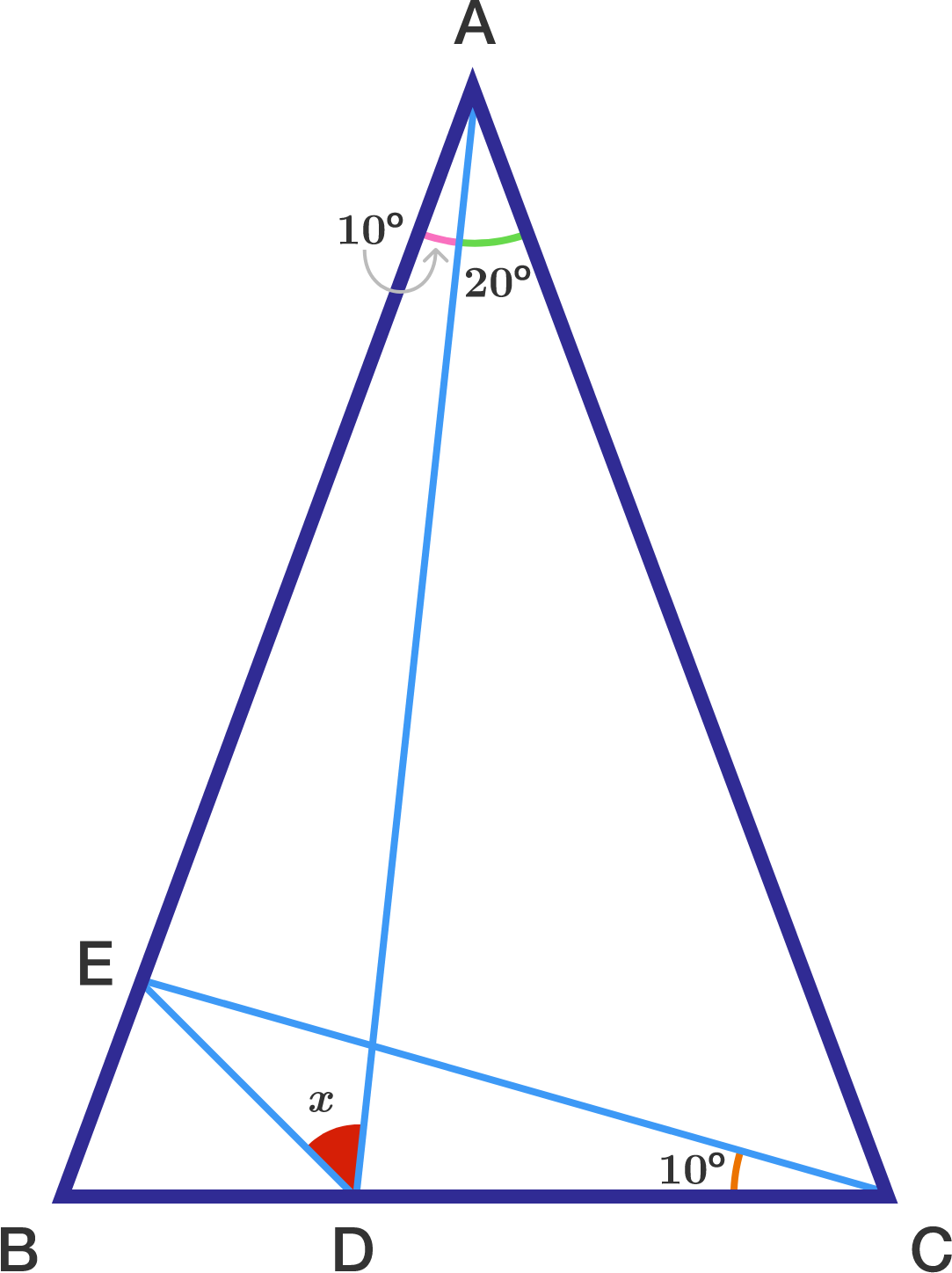# Solving Geometry In Portuguese

Geometry Level 2$\triangle ABC$ is isosceles with $AB = AC$ and $\angle BAC = 30°$. It is given that $\angle BAD = \angle B CE =10°$. Find $\angle EDA$ in degrees.

×